# How to Check If the First Letter is capitalized

This post explains that how to check if the first letter in a cell is capitalized or not in excel. How to write an excel Macro to check if the first letter in a specified cell is capitalized or not. How to test if the first letter in a table cell is capitalized with VBA macro in excel. How to capitalize the first letter in a cell with Excel formula.

## Check if the first letter is or not capitalized

To check if the first letter in a text string is or not capitalized, you can write an Excel macro to achieve it. So you can follow the below steps to check the first letter:

1# click on “Visual Basic” command under DEVELOPER Tab.2# then the “Visual Basic Editor” window will appear.

3# click “Insert” ->”Module” to create a new module4# paste the below VBA code into the code window. Then clicking “Save” button.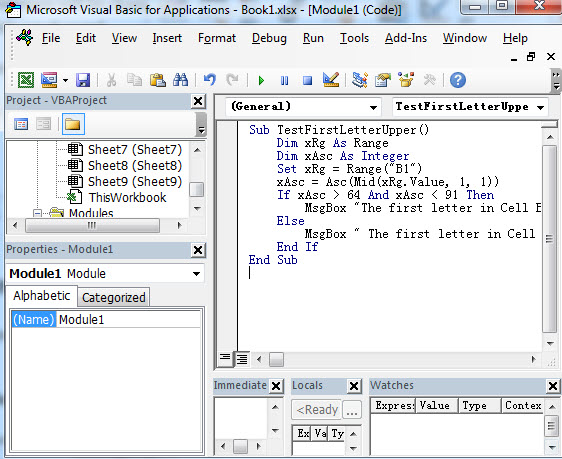```Sub TestFirstLetterUpper()
Dim xRg As Range
Dim xAsc As Integer
Set xRg = Range("B1")
xAsc = Asc(Mid(xRg.Value, 1, 1))
If xAsc > 64 And xAsc < 91 Then
MsgBox "The first letter in Cell B1 is capitalized."
Else
MsgBox " The first letter in Cell B1 is not capitalized "
End If
End Sub```

This VBA code will check if the first letter in Cell B1 is capitalized or not. So you just need to change it to other cells or range as you need.

Or you can use another VBA Macro to achieve the same result:

```Sub TestFirstLetterUpper2()
var1 = "B1"
If UCase(Left(var1, 1)) = Left(var1, 1) Then
MsgBox "First letter capitalized"
Else
MsgBox "First letter not capitalized"
End If
End Sub```

5# back to the current worksheet, then run the above excel macro.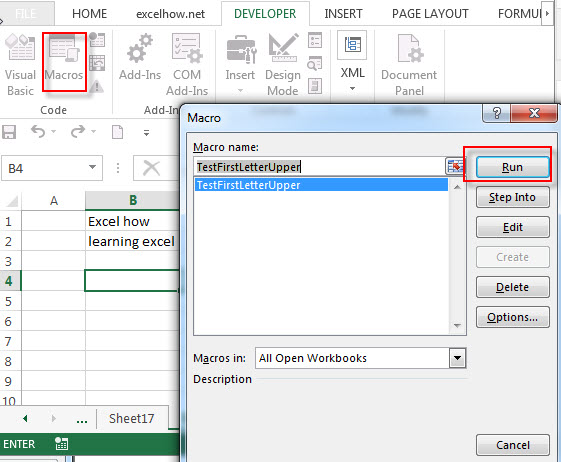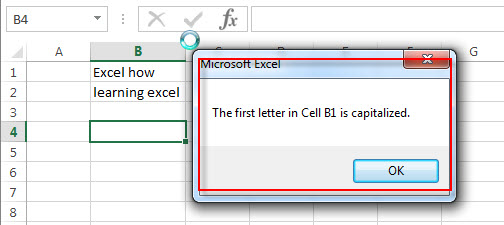## Capitalize the first letter in a Cell

Normally, it is very easy to capitalize all letters in a cell in excel, you just need to use the PROPER function to convert it.

`=PROPER(B1)`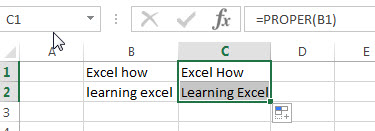If you want to capitalize only the first letter in a cell, you can create an excel formula based on the REPLACE function, the UPPER function and the LEFT function.

Assuming that you’d like to capitalize the first letter in a first word in Cell B1, then you can write down the following formula:

`=REPLACE(B1,1,1, UPPER(LEFT(B1,1)))`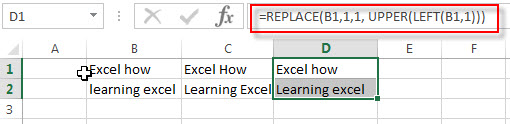You can also use another excel formula to achieve the same result as follows:

`=CONCATENATE(UPPER(LEFT(B1,1)), RIGHT(B1,LEN(B1)-1))`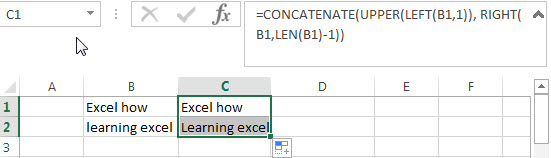### Related Functions

• Excel LEN function
The Excel LEN function returns the length of a text string (the number of characters in a text string).The LEN function is a build-in function in Microsoft Excel and it is categorized as a Text Function.The syntax of the LEN function is as below:= LEN(text)…
• Excel RIGHT function
The Excel RIGHT function returns a substring (a specified number of the characters) from a text string, starting from the rightmost character.The syntax of the RIGHT function is as below:= RIGHT (text,[num_chars])…
• Excel Replace function
The Excel DATE function returns the serial number for a date.The syntax of the DATE function is as below:= DATE (year, month, day) …
• Excel LEFT function
The Excel LEFT function returns a substring (a specified number of the characters) from a text string, starting from the leftmost character.The LEFT function is a build-in function in Microsoft Excel and it is categorized as a Text Function.The syntax of the LEFT function is as below:= LEFT(text,[num_chars])…t)…
• Excel UPPER function
The Excel UPPER function converts all characters in text string to uppercase.The UPPER function is a build-in function in Microsoft Excel and it is categorized as a Text Function.The syntax of the UPPER function is as below:= UPPER (text)…
• Excel Proper Function
The Excel PROPER function capitalizes the first character in each word of a text string and set other characters to lowercase. The syntax of the PROPER function is as below:= PROPER  (text)…
• Excel Concat function
The excel CONCAT function combines 2 or more strings or ranges together.This is a new function in Excel 2016 and it replaces the CONCATENATE function.The syntax of the CONCAT function is as below:=CONCAT (text1,[text2],…)…